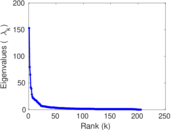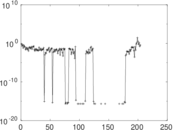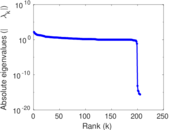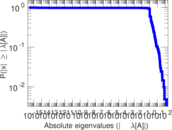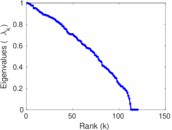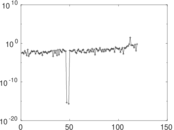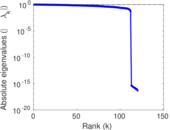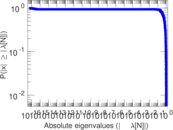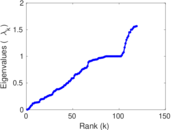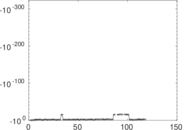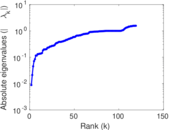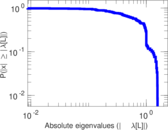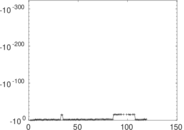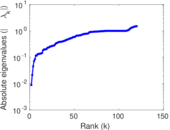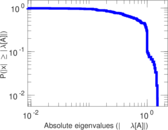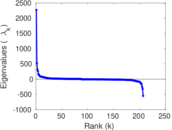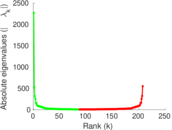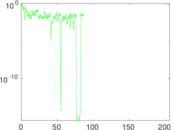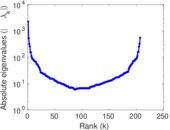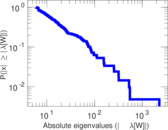# Wiktionary edits (mi)

This is the bipartite edit network of the Maori Wiktionary. It contains users and pages from the Maori Wiktionary, connected by edit events. Each edge represents an edit. The dataset includes the timestamp of each edit.

 Code `mmi` Internal name `edit-miwiktionary` Name Wiktionary edits (mi) Data source http://dumps.wikimedia.org/ AvailabilityDataset is available for download Consistency checkDataset passed all tests Category Authorship network Dataset timestamp 2017-10-20 Node meaning User, article Edge meaning Edit Network formatBipartite, undirected Edge typeUnweighted, multiple edges Temporal dataEdges are annotated with timestamps

## Statistics

 Size n = 2,262 Left size n1 = 207 Right size n2 = 2,055 Volume m = 8,909 Unique edge count m̿ = 5,029 Wedge count s = 1,269,199 Claw count z = 346,363,195 Cross count x = 80,987,469,784 Square count q = 875,409 4-Tour count T4 = 12,090,566 Maximum degree dmax = 3,190 Maximum left degree d1max = 3,190 Maximum right degree d2max = 120 Average degree d = 7.877 10 Average left degree d1 = 43.038 6 Average right degree d2 = 4.335 28 Fill p = 0.011 822 2 Average edge multiplicity m̃ = 1.771 53 Size of LCC N = 1,871 Diameter δ = 15 50-Percentile effective diameter δ0.5 = 3.326 72 90-Percentile effective diameter δ0.9 = 5.894 71 Median distance δM = 4 Mean distance δm = 3.997 51 Gini coefficient G = 0.710 245 Balanced inequality ratio P = 0.235 436 Left balanced inequality ratio P1 = 0.092 266 2 Right balanced inequality ratio P2 = 0.320 126 Relative edge distribution entropy Her = 0.768 012 Power law exponent γ = 2.290 54 Tail power law exponent γt = 1.731 00 Tail power law exponent with p γ3 = 1.731 00 p-value p = 0.126 000 Left tail power law exponent with p γ3,1 = 1.631 00 Left p-value p1 = 0.218 000 Right tail power law exponent with p γ3,2 = 4.211 00 Right p-value p2 = 0.015 000 0 Degree assortativity ρ = −0.090 078 1 Degree assortativity p-value pρ = 1.560 35 × 10−10 Spectral norm α = 152.889 Algebraic connectivity a = 0.008 784 51 Spectral separation |λ1[A] / λ2[A]| = 1.915 17 Controllability C = 1,699 Relative controllability Cr = 0.810 205

## Plots

### Fruchterman–Reingold graph drawing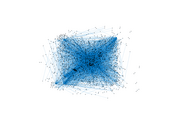### Degree distribution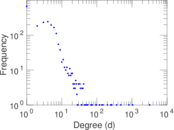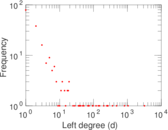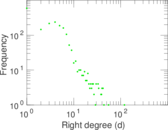### Cumulative degree distribution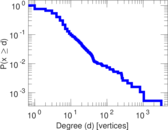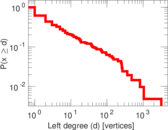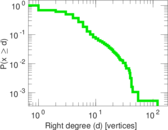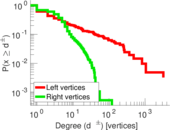### Lorenz curve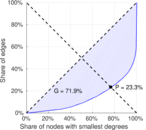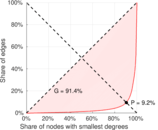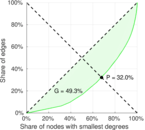### Spectral distribution of the adjacency matrix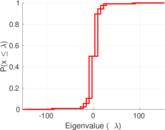### Spectral distribution of the normalized adjacency matrix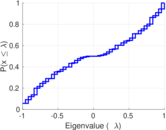### Spectral distribution of the Laplacian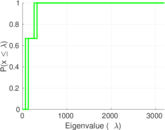### Spectral graph drawing based on the adjacency matrix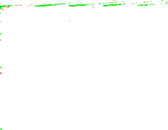### Spectral graph drawing based on the Laplacian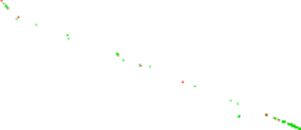### Spectral graph drawing based on the normalized adjacency matrix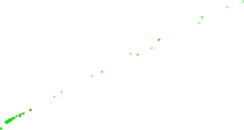### Degree assortativity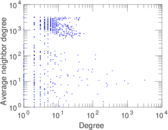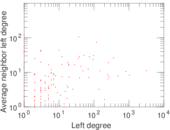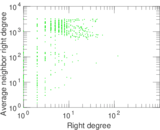### Zipf plot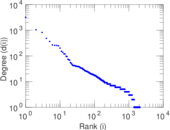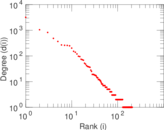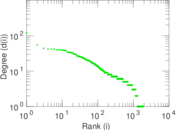### Hop distribution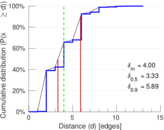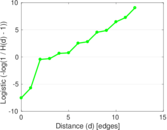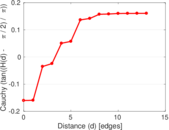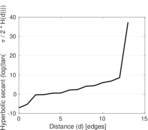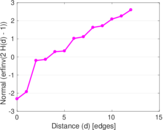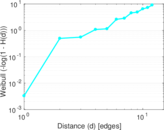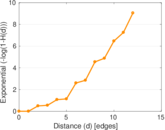### Double Laplacian graph drawing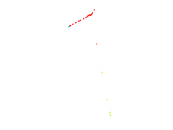### Delaunay graph drawing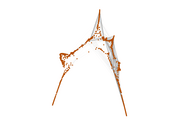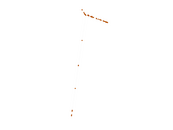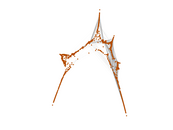### Edge weight/multiplicity distribution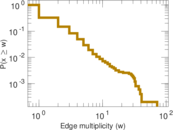### Temporal distribution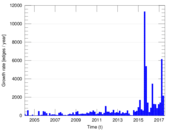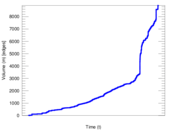### Temporal hop distribution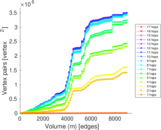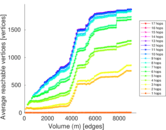### Diameter/density evolution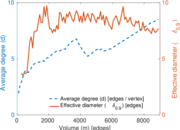### Matrix decompositions plots